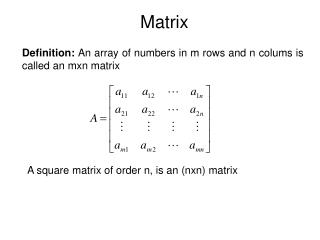DownloadDownload PresentationMatrix

# Matrix

Télécharger la présentation## Matrix

- - - - - - - - - - - - - - - - - - - - - - - - - - - E N D - - - - - - - - - - - - - - - - - - - - - - - - - - -
##### Presentation Transcript

1. Matrix Definition: An array of numbers in m rows and n colums is called an mxn matrix A square matrix of order n, is an (nxn) matrix

2. For example, since both matrices are of order 2x2 and all corresponding entries are equal. Matrix Equality Two matrices A = [aij] and B = [bij] are equal if they have the same order and aij= bijfor every i and j. .

3. Matrix Addition Definition: The sum of two matrices is the matrix where each element is the sum of the corresponding elements of the two matrices. NOTE: Matrix addition is only defined when the matrices have the same dimension. Examples:

4. Matrix Substraction Definition: The substraction of two matrices is the matrix where each element is the difference of the corresponding elements of the two matrices. NOTE: Matrix substraction is only defined when the matrices have the same dimension. Example:

5. Matrix Addition Theorem 1: Let A, B, and C be matrices of the same size, then Commutative law of addition Associative law of addition

6. Definition:Let a be a number and A=(aij)mxn be any matrix. Then This means that aA is the matrix by multiplying each entry of A by the same number a Scalar Multiplication

7. Definition:Let A=(aij) be a mxnmatrix. The negative of the matrix A is the mxn matrix B=(bij) such that bij=-aij for all i,j. The negative of A is written as –A. For example, Negative of a Matrix

8. Definition:The mxnmatrix in which every entry is 0 is called the mxn zero matrix and is denoted by 0mxn or simply 0. We note that A+(-A)=0, the zero matrix. We usualy write A+(-B) as A-B Zero Matrix

9. Multiplication of Matrices • The product of two matrices A and B is defined if A is mxn and B is nxp • We first give the rule for multplication in special case when A is 1xn and B is nx1 Then their product AB is defined to be a 1x1 matrix,

10. Multiplication of Matrices Now we give the rule for multiplication of matrices in general. Definition: Let A=(aij)mxn and B=(bij)nxq. Then AB =(cij)mxp

11. Multiplication of Matrices     10 12 20 22 30 32 1 2 3 4 5 6    1*10+2*20+3*30 1*12+2*22+3*32 4*10+5*20+6*30 4*12+4*22+6*32 # of Columns of A must = # of Rows of B

12. Diagonal of a Matrix Definition: If A is an nxn matrix, then the line joining (1,1) entry, (2,2) entry,…,(n,n) entry is called the diagonal (or the main diagonal) of the matrix. Definition: Any nxn matrix D is called diagonal matrix if each entry not on the diagonal is 0. For example,

13. Identity Matrix Definition: An nxn matrix A=(aij), where aij=1 whenever i=j, and aij=0 whenever is called the identity matrix of order n. And it is denoted by Square matrix with ones on the diagonal and zeros elsewhere. It can be easily proved that if A is an mxn matrx, then

14. Properties of Matrix Multiplication

15. Transpose Definition: Given an mxn matrix A, the transpose of A is the nxm matrix, denoted by AT, whose columns are formed from the corresponding rows of A. In other words, the ith row of AT is the ith column of A for all i. Example: Let What is AT ?

16. Rules related to transpose:

17. Matrix Powers Recall that matrix multiplication is associative, i.e. if A, B and C have the proper dimensions, then A ( BC ) = ( AB ) C, so the parentheses are unnecessary and the product can be written as ABC. If A is an n x n matrix and p is a positive integer, can define Again, if A is an n x n matrix, adopt the convention

18. Triangular Matrices An n x n matrix A= [ aij ] is called upper triangular if aij= 0 for i > j An n x n matrix A= [ aij ] is called lower triangular if aij= 0 for i < j Note: A diagonal matrix is both upper and lower triangular The n x n zero matrix is both upper and lower triangular

19. Symmetry Defn - A matrix A is called symmetric if AT=A Defn - A matrix A is called skew-symmetric if AT=-A Comment - If A is skew-symmetric, then the diagonal elements of A are zero Comment - Any square matrix A can be written as the sum of a symmetric matrix and a skew-symmetric matrix